# Unifix Cube Template PrintableUpdated on Dec 01, 2021By Printablee Team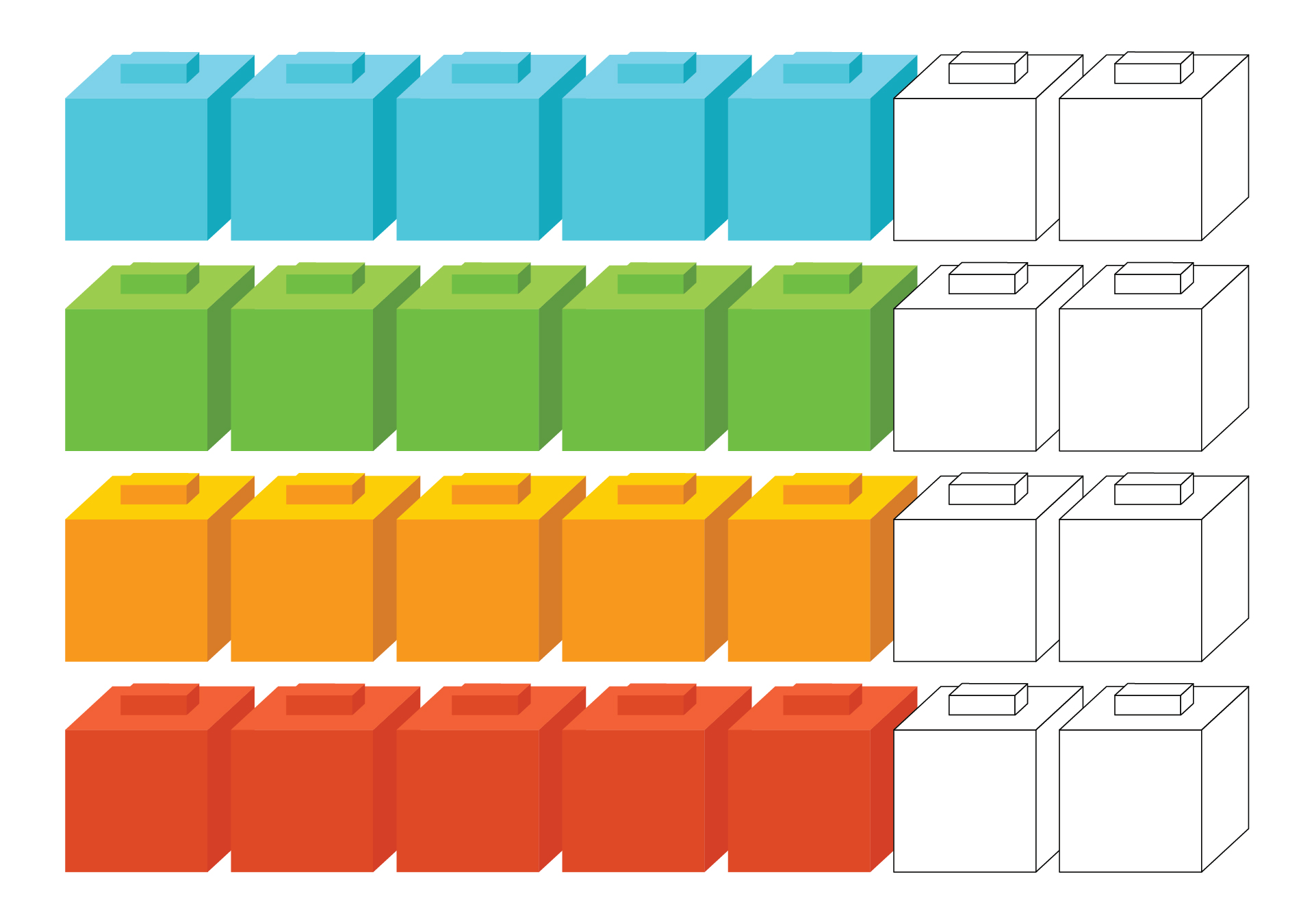### What are the Unifix Cubes?

Unifix cubes are colorful interlocking cubes that are used to help children learn math concepts. Each cube represents one unit, and each cube has an opening on one side that can be connected to other cubes. Unifix cubes are usually used on kindergarten and lower elementary grades to practice basic math operations such as addition, subtraction, multiplication along with counting, sorting, and comparing. Unifix cube can help learning activities more fun and easier especially for kids with learning difficulties.

### How to Use Unifix Cubes?

There are so many learning activities we can do using unifix cubes.
1.    Make a Number. Choose a number and ask children different ways to make that number. For example, to make 1, children can use five red cubes and five green cubes, nine red cubes, and one yellow cube, or, six blue cubes and four yellow cubes.
2.    Subtraction. Use a small collection of unifix cubes. Color doesn't matter in this activity. Ask the children to count the cubes on the table, and then ask them to close their eyes. Hide some other cubes, and ask the children to count how many cubes left on the table and guess how many cubes that are hidden. This will help children to visualize the basic concept of subtraction.
3.    Measurement. Unifix cubes can be connected and create a line. This can be a perfect tool to use on measuring activity. Use a variety of objects to measure with the cubes.
4.    Comparing numbers. Ask children to build a number using unifix cubes using the same color. And then ask them to build another number using a different color. Line them up side by side and the children will compare and understand that longer cubes are the higher number.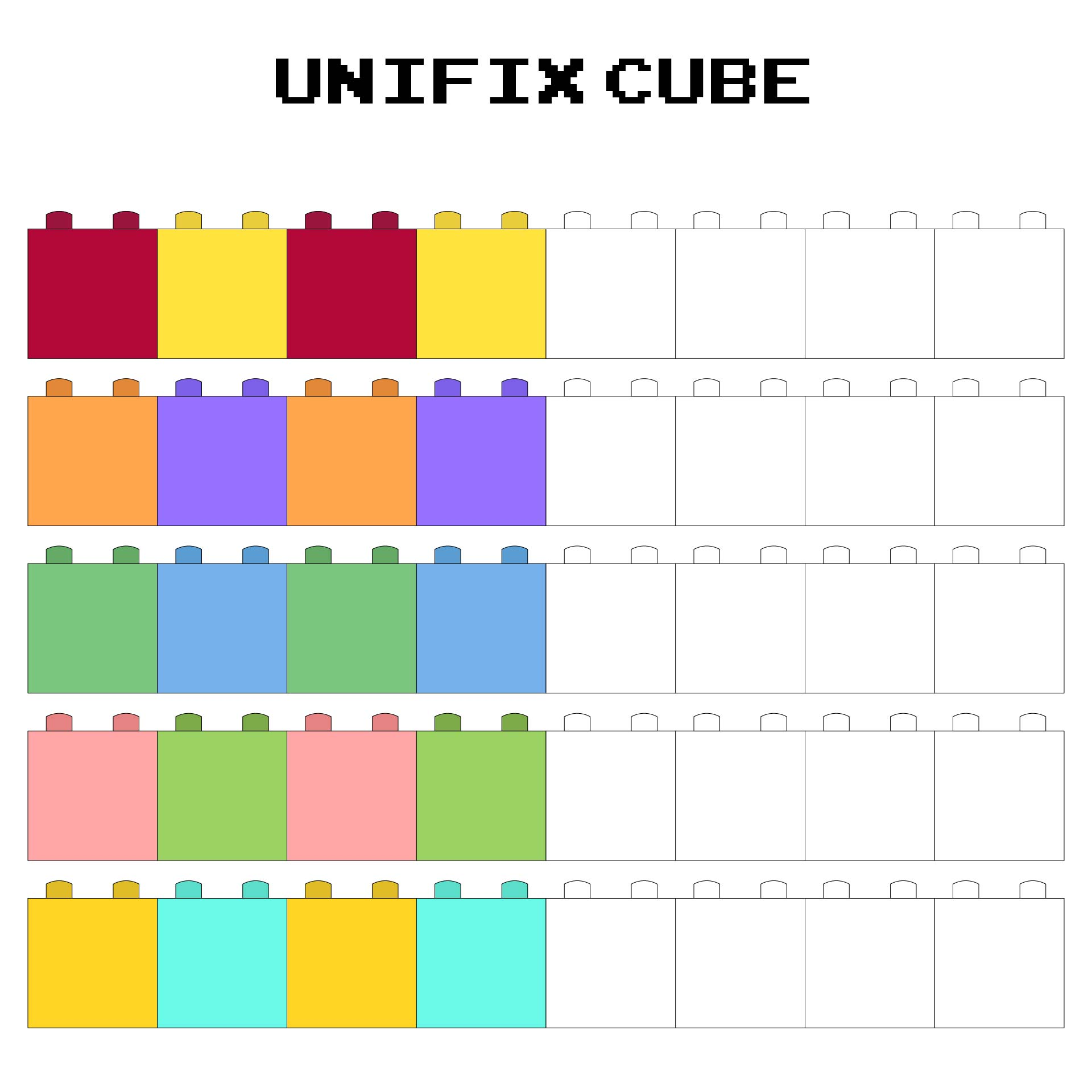We also have more printable template you may like:
Chevron Printable Label Templates
Lego Block Printable Templates
Tool Box Printable Template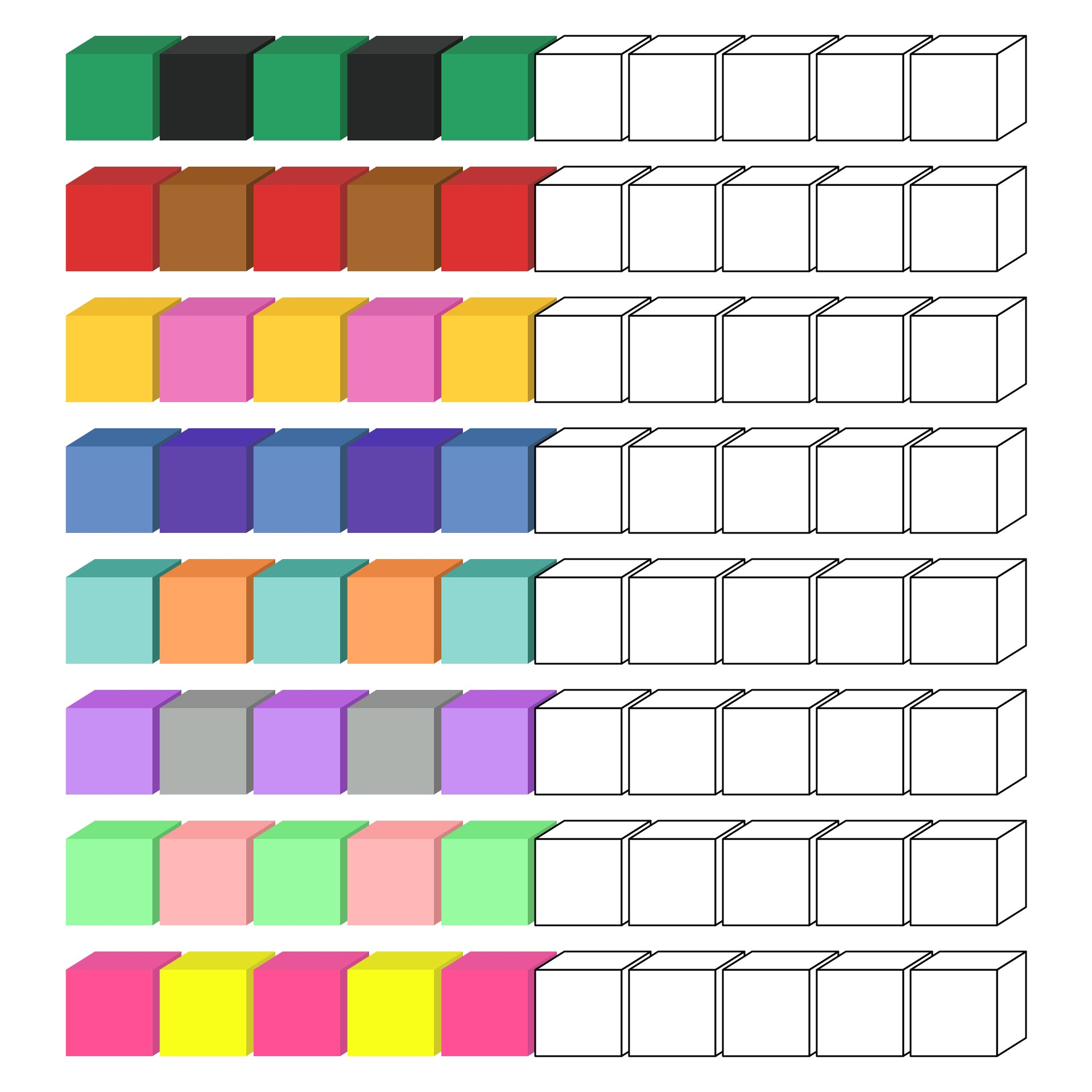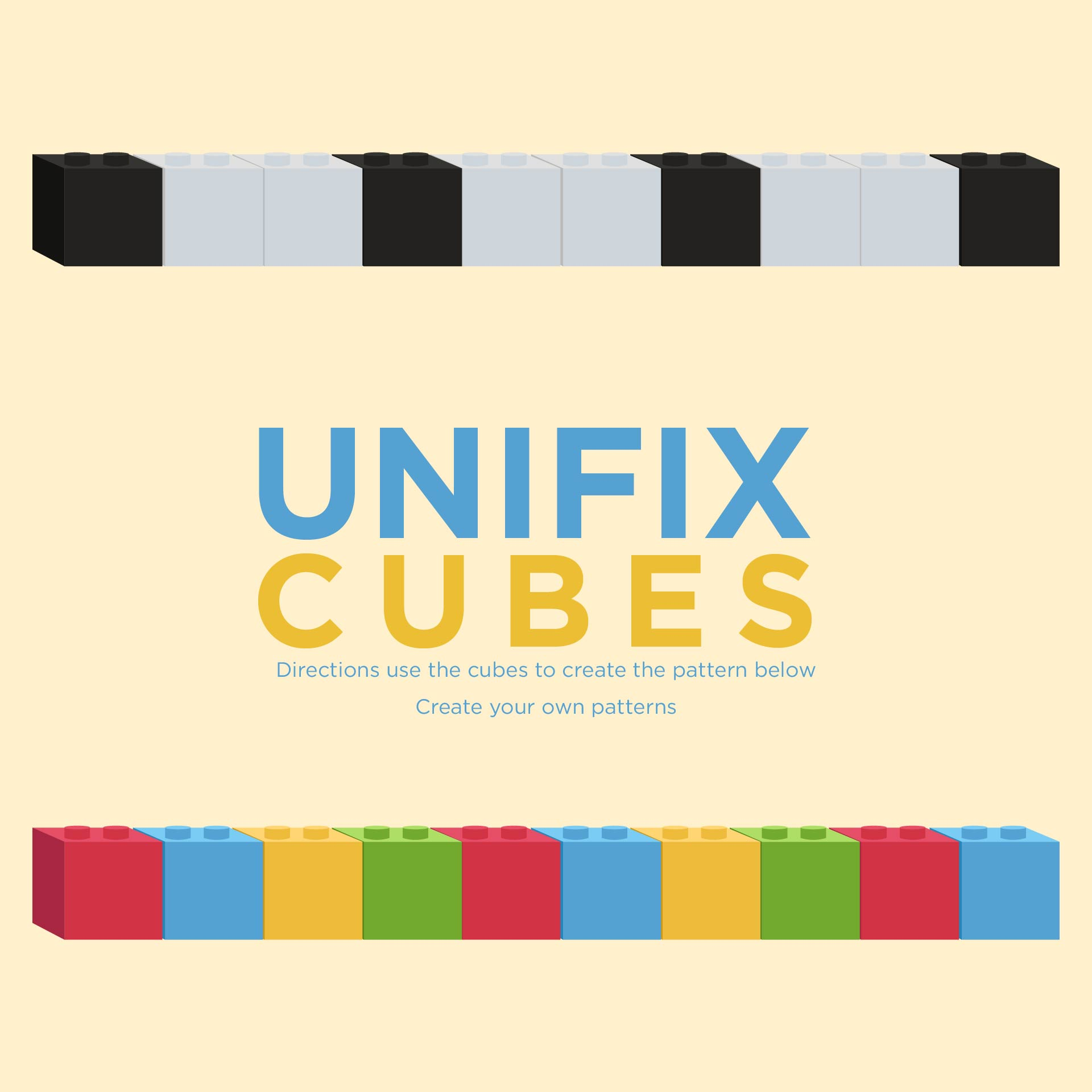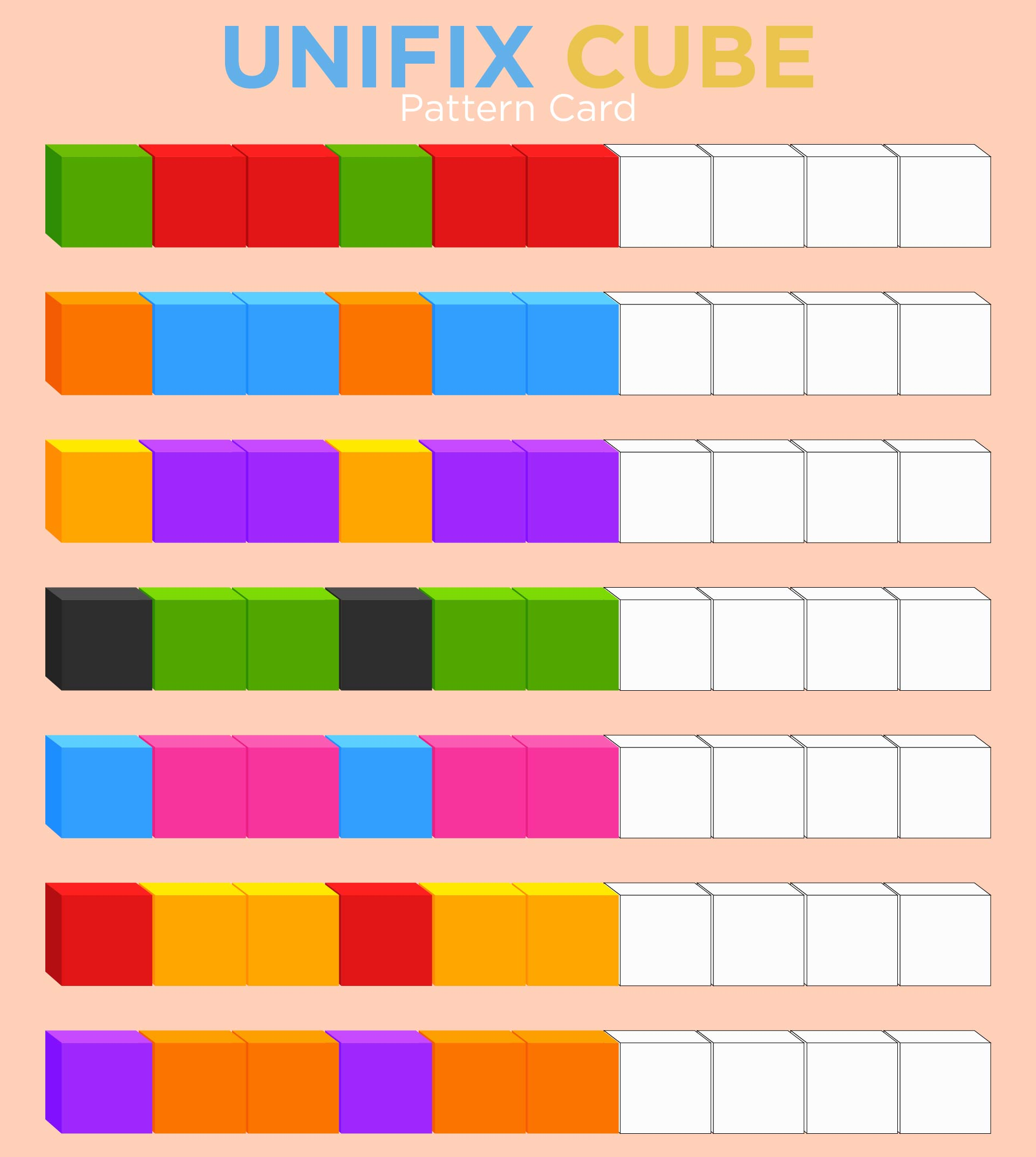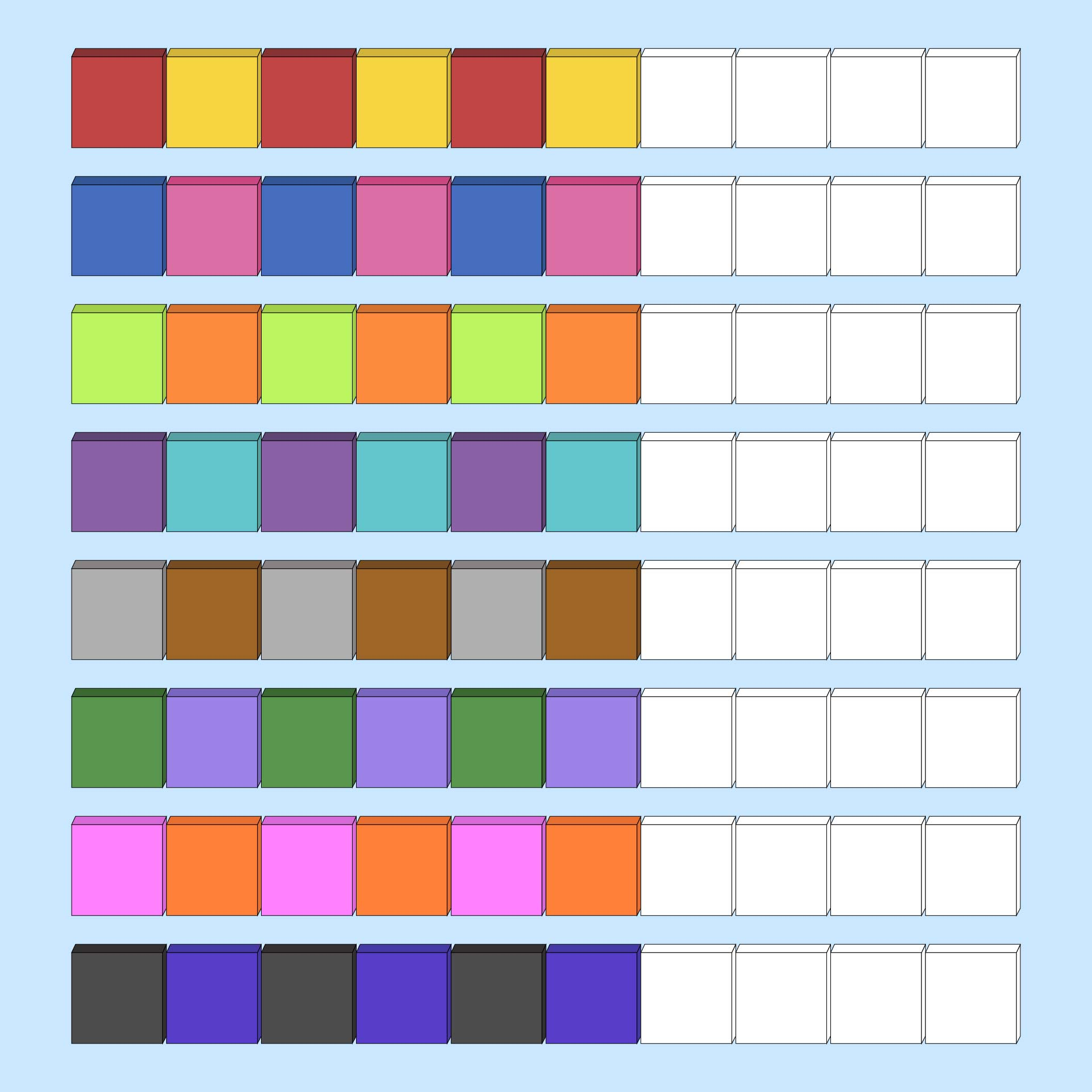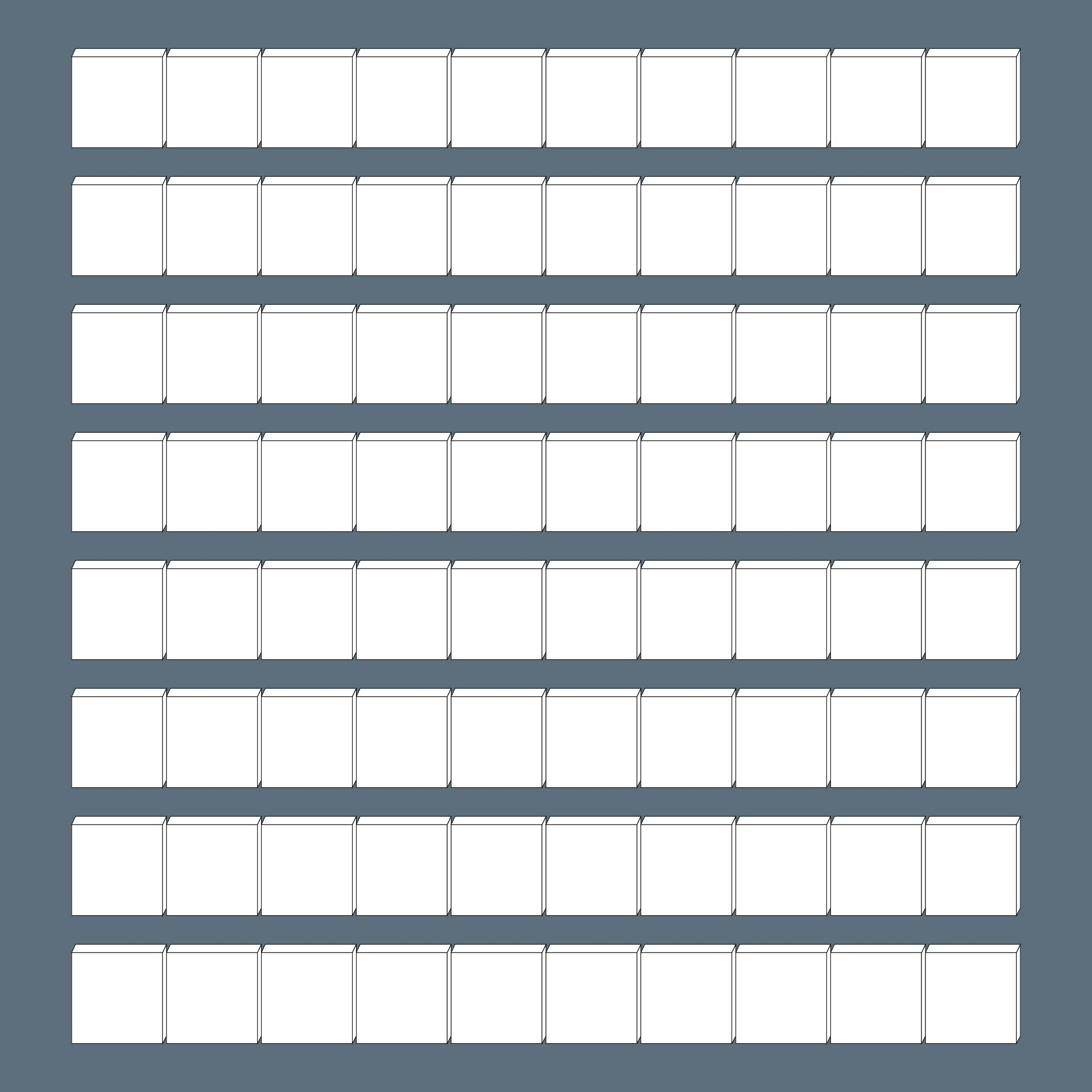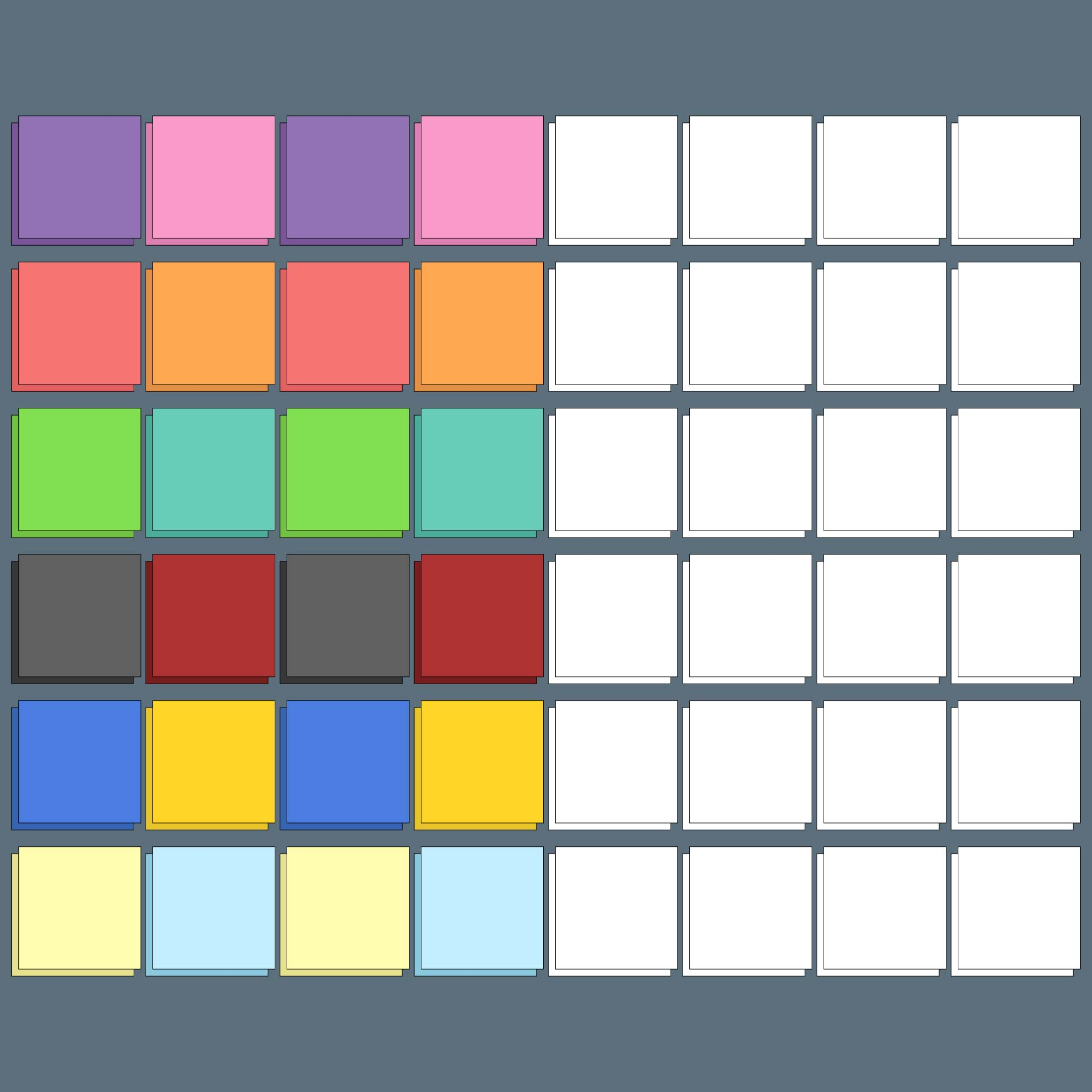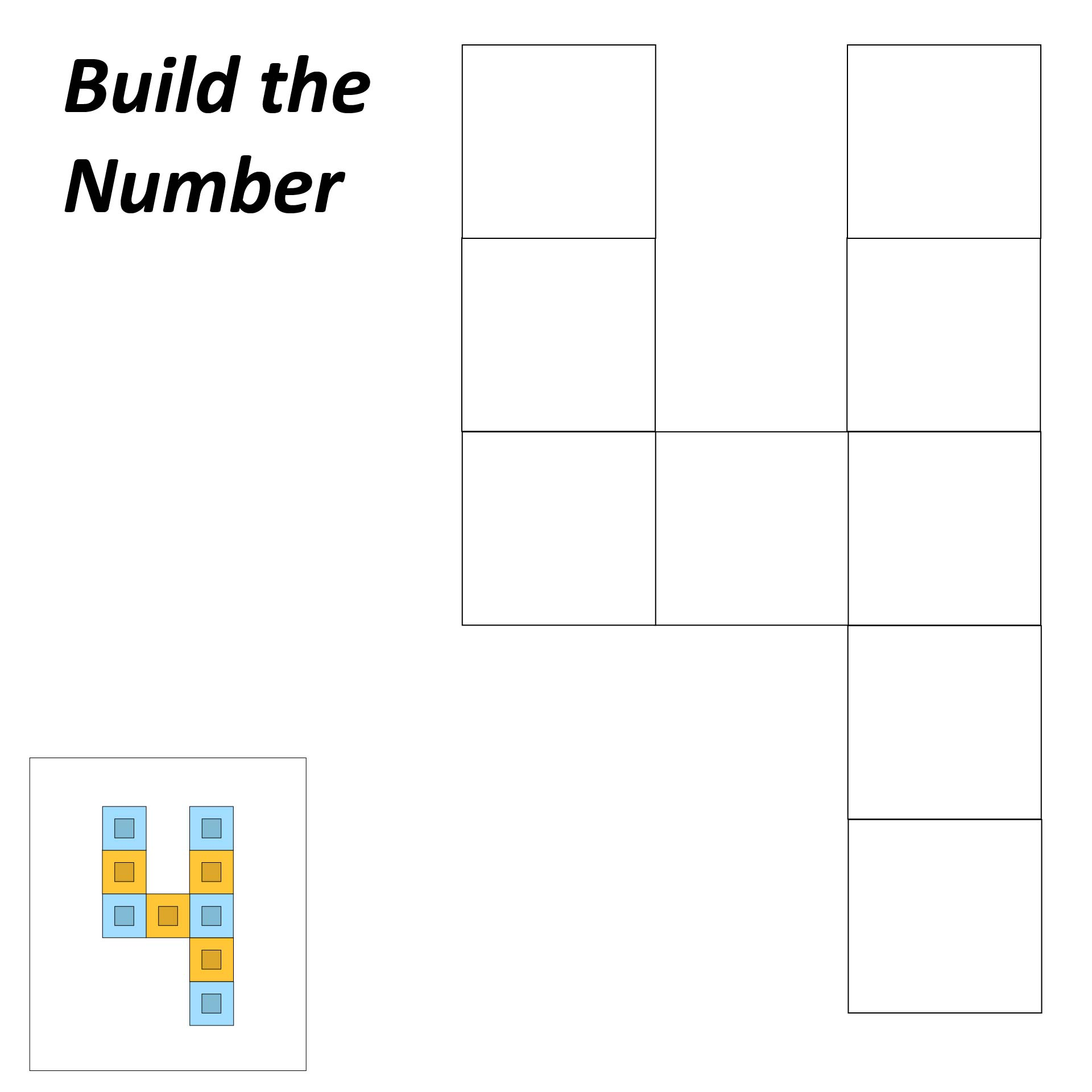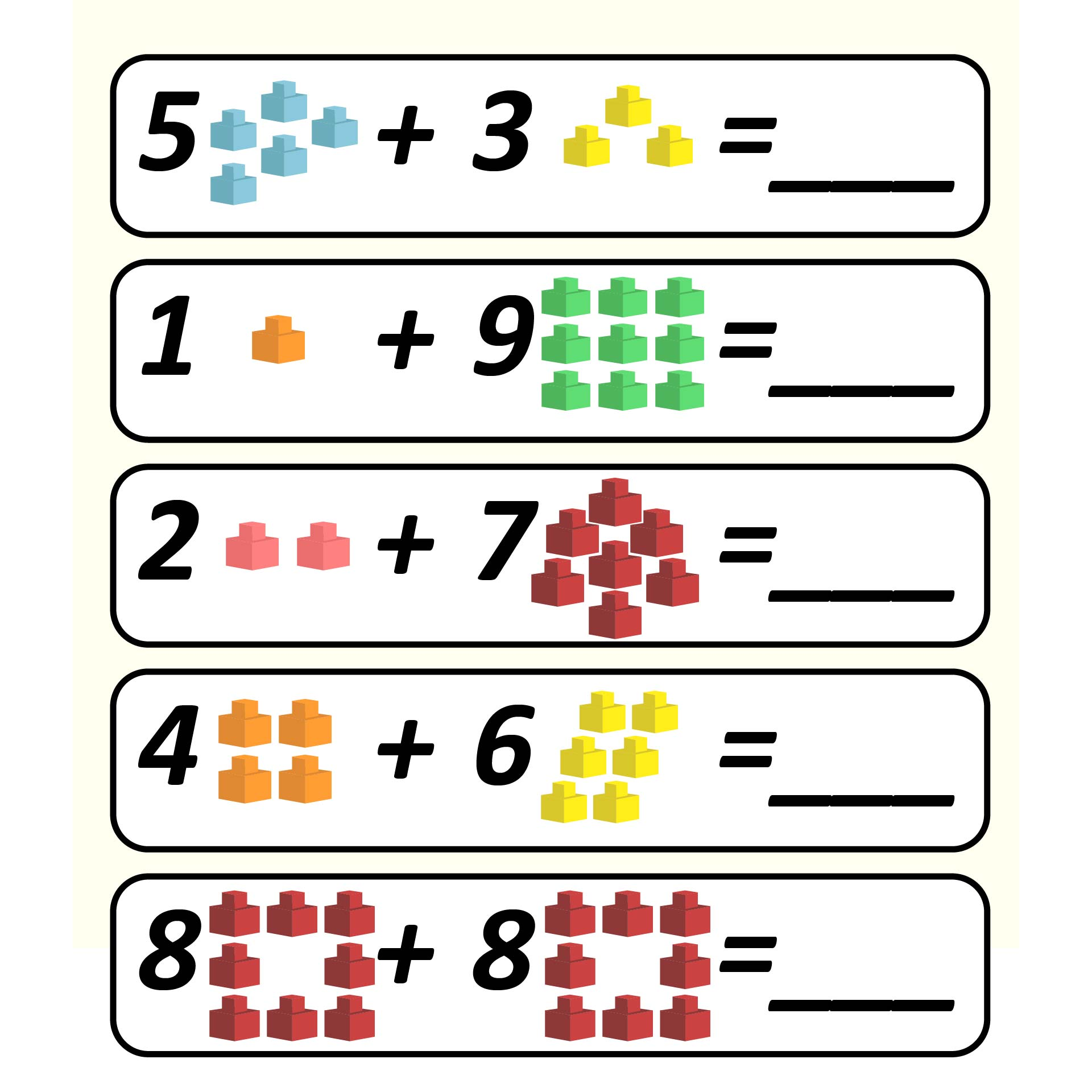### How do Unifix Cubes help Students?

Unifix cubes help students develop spatial sense. They are also used to develop an understanding number and measurement concepts. Students can use unifix cubes to create, identify, and extend patterns. The variety of colors also allows children to use the cubes in probability experiments.
For example, children can use unifix cubes to build a cube train, and then you can ask them what color is the tenth train? This will help children to count. Children also can use the cubes to create simple squares with different patterns. For example, use different colors of nine cubes into a simple square. Unifix cubes are great tools to learn and can be used to teach teamwork as kids can use the cubes in groups.